# Change the numbers in the ratio

Change the numbers 29, 38, and 43 in a 3: 4 ratio.

a =  21.75
b =  28.5
c =  32.25

### Step-by-step explanation: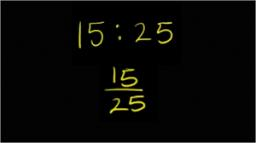Did you find an error or inaccuracy? Feel free to write us. Thank you!Tips to related online calculators
Need help to calculate sum, simplify or multiply fractions? Try our fraction calculator.
Check out our ratio calculator.

## Related math problems and questions:

• The rawThe raw data presented here are the scores (out of 100 marks) of a market survey regarding the acceptability of new product launched by a company for a random sample of 50 respondents: 40 45 41 45 45 30 30 8 48 25 26 9 23 24 26 29 8 40 41 42 39 35 18 25 3
• PamelaLara Pamela spends her salary of 3000 for food, clothing, recreation and savings, which are in the ratio of 48:20:15:37, respectively. How much does he spend for savings?
• Photo egative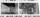Negative dimensions are 36mm and 28mm. What will be the photo size in the 21:4 ratio?
• Reducing scale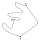I am sizing some landscaping and I measured everything for a 20 to 1 scale. I realized when I was done that it was at 30 to 1. How can I convert what I already have to 30 to 1. (Example, grass area is 22,871.6' at 20 to 1, how do you figure it out to be 3
• Fractions and mixed numerals(a) Convert the following mixed numbers to improper fractions. i. 3 5/8 ii. 7 7/6 (b) Convert the following improper fraction to a mixed number. i. 13/4 ii. 78/5 (c) Simplify these fractions to their lowest terms. i. 36/42 ii. 27/45 2. evaluate the follow
• N percentille problemHere is a data set (n=117) that has been sorted. 10.4 12.2 14.3 15.3 17.1 17.8 18 18.6 19.1 19.9 19.9 20.3 20.6 20.7 20.7 21.2 21.3 22 22.1 22.3 22.8 23 23 23.1 23.5 24.1 24.1 24.4 24.5 24.8 24.9 25.4 25.4 25.5 25.7 25.9 26 26.1 26.2 26.7 26.8 27.5 27.6 2
• Ratio6 numbers are in the ratio 1:5:1:5:5:5. Their sum is 242. What are the numbers?
• GardenThe rectangular garden has dimensions of 27 m and 30 m. Peter and Katka split it in a ratio of 4:5. How many square meters did Katkin measure part of the garden?
• Ornamental shrubsChildren committed to planting 240 ornamental shrubs. Their commitment, however, exceeded by 48 shrubs. Write the ratio of actually planted shrubs and commitment by lowest possible integers a/b.
• BuildingAt the building, we divided 240 boards into two piles in a 5: 3 ratio. How many were fewer boards in the lower pile?
• Ratio three numbers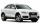Three numbers SUV are in the ratio 1:2:3. Their sum is 24. Find this numbers and write their add and sum.
• Report 2A School reports students to teacher ratio of 6:1. If there are 45 teachers in the School, how many students are there?
• FortyForty five of the 80 students were girls. What is the ratio of girls to boys?
• Unknown number 5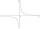Daniel thinks an integer. When he change this number at a ratio of 2:5, he got the number 2.8. Determine what number think Daniel.
• Two brothersThe two brothers were to be divided according to the will of land at an area of 1ha 86a 30m2 in a ratio of 5:4. How many will everyone get?
• Ratio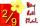Write the ratio with other numbers so that the value is the same: 2: 9
• Moneys in triple ratioMilan, John and Lili have a total 344 euros. Their amounts are in the ratio 1:2:5. Determine how much each of them has?